American Journal of Software Engineering and Applications
Volume 4, Issue 3, June 2015, Pages: 50-55

Local Feature Extraction Models from Incomplete Data in Face Recognition Based on Nonnegative Matrix Factorization

Yang Hongli1, *, Hu Yunhong2

1Science College, Shandong University of Science and Technology, Qingdao, Shandong, P. R. China

2Applied Mathematics Department, Yuncheng University, Yuncheng, P. R. China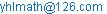(Yang Hongli)

Yang Hongli, Hu Yunhong. Local Feature Extraction Models from Incomplete Data in Face Recognition Based on Nonnegative Matrix Factorization. American Journal of Software Engineering and Applications. Vol. 4, No. 3, 2015, pp. 50-55. doi: 10.11648/j.ajsea.20150403.12

Abstract: Data missing usually happens in the process of data collection, transmission, processing, preservation and application due to various reasons. In the research of face recognition, the missing of image pixel value will affect feature extraction. How to extract local feature from the incomplete data is an interesting as well as important problem. Nonnegative matrix factorization (NMF) is a low rank factorization method for matrix and has been successfully used in local feature extraction in various disciplines which face recognition is included. This paper mainly deals with this problem. Firstly, we classify the patterns of image pixel value missing, secondly, we  provide the local feature extraction models basing on nonnegative matrix factorization under different types of missing data, thirdly, we compare the local feature extraction capabilities of the above given models under different missing ratio of the original data. Recognition rate is investigated under different data missing pattern. Numerical experiments are presented and conclusions are drawn at the end of the paper.

Keywords: Local Feature Extraction, Incomplete Data, Face Recognition, NMF, Model

1. Introduction

Data missing often happens in the process of data collection, transmission, processing, preservation and application due to various reasons. For example, in EEG signal acquisition process, the electrodes will cause some loss of signal data due to the fault or other reasons; satellite images data may also be lost during the transmission; during the process of the image denoising, division and segmentation, image data may be lost. There are many types missing data for an image, of which pixel values data missing is considered in this paper. When the pixel value data is lost, the corresponding pixel value matrix is called incomplete matrix. Incomplete matrix factorization and corresponding feature extraction problem has been raised for decades,the most commonly used method is principal component analysis . However, principal component analysis method is generally based on the global feature extraction by virtue of the statistical properties of the data. NMF is a low rank matrix approximation for nonnegative matrix based on a nonnegative matrix factorization. It is an effective way to obtain the local feature of the data matrix representation. Nonnegative matrix factorization model has been successfully applied to many fields, but most of these applications is for complete matrix without missing data. For incomplete matrix, research is not so much.

Weighted nonnegative matrix factorization model is pioneered by after NMF model was proposed. It was originally used to extract the characteristics of the environmental data. After the model was proposed, many different algorithms for solving this model have been given. Weighted matrix decomposition model was firstly used for the problem with missing data is but without considering nonnegative constraints;  considered the nonnegative constraints and applied it to the missing data model, more applications can be found in .  is also the first model to combine the EM algorithm and NMF algorithm, the author used model and algorithm to solve the case of testimonials with missing data. Recent research results about weighted nonnegative matrix factorization and its application is the following:  gave two new matrix factorization algorithm,  presented a semi-supervised classification model based on weighted nonnegative matrix decomposition,  studied the weighted tensor decomposition and its application, which gave an weighted tensor decomposition model to extract feature from missing data, but  did not consider the nonnegative constraints. Other studies about weighted nonnegative matrix factorization in facial feature extraction and the application are .

This paper studies the local feature extraction problem in face recognition from incomplete matrix by nonnegative matrix factorization. Three NMF models are presented in this paper: direct interpolation model, weighted nonnegative matrix factorization model, the combination of taking the mathematical expectation of the missing data (Expectation) and nonnegative matrix factorization model. The organization of the paper is as follows: inspired by , we classified the pattern of the missing data in section 2, presented three local feature extraction models for different types of missing data in section 3, compared the computation cost and the performance of different models are given in this section, in section 4 numerical experiments verified the effectiveness of several algorithms, conclusions and future research directions are given at the end of this paper.

2. Classification of the Missing Data Pattern

The classification of missing data problem was first put up by Little and Rubin in the field of the humanities. He classified the pattern of missing data in the humanities field into three categories. In the experimental science field, Giorgio and Bro also divided the missing data into three categories: random missing values (RMV in brief, which means the position of the missing data is at random); missing at random spectral values (RMS in brief), that is, the entire column or row data is missing randomly, missing system spectral values (SMS in brief). Inspired by their classification as well as the specific application background of the missing data, we divide the missing image pixel values of an image into two categories. 1) Randomly missing values(RMV for brief), in this case, the position of the missing pixel values is random, which often occurs due to the denoising of the image. 2) The missing of image data is not at random, this type of missing is divided into two categories. One is due to the faults of signal collecting equipment, which makes the data in whole rows or columns of the image matrix missed. Since this type of data missing is related to the system, it is called Systematically missing values (SMV for brief). For example, in EEG signal analysis, due to the fault of the electrodes which make the system could not collect the data successfully, so data is missing or wrong. The other is caused by the person’s choice, such as image segmentation, it requires all pixel value that below 0 be changed the same value, it is called the systematically missing spectral values (SMS for brief).

3. Model

This paper deals with the problem of local features extraction from incomplete pixel value matrix by virtue of the following nonnegative matrix factorization models.

3.1. Model 1 (Interpolation NMF Model)

If the ratio of the missing data is small (such as less than 5 percent), the usual method to restore the incomplete matrix is to in place of the missing data with some data (such as expectation), then to extract the local feature from the restored matrix. The method is called interpolation. The regular interpolation methods are such as mean imputation, regression imputation, pattern matching . When the ratio of the missing data is large, the interpolation method does not fit in the reason that the error between the restored matrix and the former matrix is large. The feature obtained by the NMF methods is the local feature, the missing data means the missed local feature. If we ignore the effect of missing data and are only interested in the available data, the local feature can be obtained by weighted NMF method (model 2).

3.2. Model 2 (Weighted Nonnegative Matrix Factorization Model(WNMF))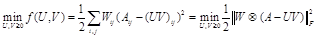in which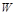is a (0,1)matrix,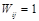if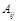is not missing, otherwise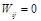, "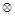" is the Hadamard product of the matrix. Model 2 becomes the usual complete NMF model as all the entries of matrixare 1. When the entries ofis 0, it means the missing of the element at the position of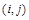: The effect for the objective function is 0, so model 2 does not take into account the missing data. For the unconstrained minimization problem, the first order optimality condition is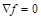,We note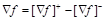, in which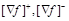is the positive and negative part respectively with positive elements, then we can reformulate NMF iteration formula as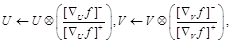and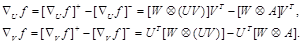Then the iteration updates can be reformulate as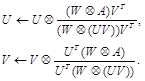The above model has the same form of the iteration update as the update that in , it is called weighted NMF model (WNMF for brief).

Remark: There are some difference between Model 1 and Model 2. Model 1 uses the interpolation method to restore the missing data matrix before iteration updates, all the elements of the matrix are involved in the operation, while in model 2, the missing data does not participate in the operation.

3.3. Model 3 (EM-NMF Model)

Paper presented a local feature extraction model based on a modified NMF algorithm for the matrix with missing data(called EM-NM algorithm). We use the idea and the algorithm for the local feature extraction in face recognition with incomplete data in this paper. In the proposed model, EM algorithm estimates the unknown parameters generally involves two steps: taking expectation (Expectation Step) and solving maximization (Maximum Step). The method utilizes the idea of maximum likelihood estimation. Firstly, it classifies the data of the matrix into not missing data and missing data, takes the missing data as unknown parameters. In the first step, it selects the initial value of unknown parameters, calculates the parameter by taking mathematical expectation of the unknown parameters. The second step is to use the missing data as the predicted value of the maximum likelihood function of the unknown parameters obtained by the new estimate, repeating the above procedure until a solution satisfies the conditions to be obtained. It needs more computational cost in the process expectation maximization. Paper gave an EM-based standard NMF approach, numerical experiments showed that results of EM-NMF method significantly better than results obtained by the WNMF method. The algorithm step is as follows.

E-step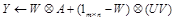M-step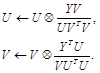in which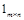is a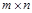size matrix with all entries are 1. The above model is called expectation maximize nonnegative factorization(EMNMF for brief).

3.4. The Computational Cost of Models

The computational cost of the above three models is different in the reason that the iteration updates of the algorithms is not the same. If the data matrix A issize, WNMF algorithm will cost more 4mn multiplication operation in each iteration than the standard NMF. EMNMF algorithms has an additive computation of taking expectation in each iteration, which will have 2mn more multiplication operation than WNMF model, if the iteration times for E-step and M-step is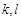respectively, EMNMF will has more 2lmn multiplication operation than WNMF algorithm, and will have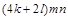multiplication operation more than that of NMF.

4. Numerical Experiments

Four experiments are given in this paper. The database we used is the Standards ORL face Database for face recognition. The size of the matrix is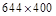. The aim of Experiment 1 to Experiment 3 is to test the performance of three models in local feature extraction aspect. We test these models with three type of data missing(Experiment 1 for RMV, Experiment 2 for SMV, and Experiment 3 for SMS), the criterion of the performance is iteration times, iteration time and the approximation error between matrix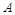and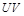. We also consider the local feature error between the original and the results we obtained, as well as the sparseness of the extracted local feature. The aim of Experiment 4 is to test the recognition rate by virtue of the local feature obtained. In model 1,we use 0 to fill in the missing data, the stopping criterion for the iteration is the error between continuous two iteration is less than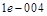.We use the same initial value for different models in order to avoid the different local optimizer of algorithms.

4.1. Experiment 1

4.1.1. Experimental Results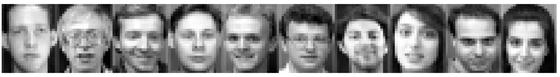(a) some of original images from ORL Database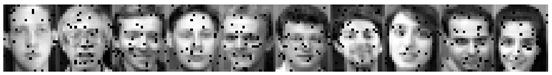(b) some of images with 5% RMV missing data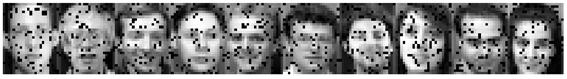(c) some of images with 10% RMV missing data

Figure 1. (a)some of original images from ORL Database,(b)some of images with 5% RMV missing data,(c)some of images with 10% RMV missing data.

Table 1. Experimental results about time, iteration, error with RMV missing data.

 Original image（data not missing） 5% data missing Time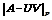Iteration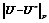TimeIterationModel 1 132.07 51.0778 3120 _ 93.11 76.8583 2200 0.1971 Model 2 _ _ _ _ 114.77 51.4471 2714 0.1202 Model 3 _ _ _ _ 159.51 50.0011 2828 0.0939
 10% data missing 20%data missing TimeI IterationTimeIterationModel1 74.41 92.7477 1757 0.1791 65.42 113.8371 1543 0.2220 Model 2 185.70 51.2849 4355 0.1585 140.74 51.7154 3326 0.1919 Model 3 148.27 48.6117 2626 0.1675 193.98 46.1209 3427 0.1971
 40% data missing 70 % data missing TimeIterationTimeIterationModel1 59.54 132.6979 1402 0.2626 59.15 137.9275 1392 0.3012 Model2 89.87 52.1856 2124 0.1559 80.41 53.3961 1899 0.1682 Model3 156.03 41.2126 2746 0.1632 156.90 34.8928 2759 0.1679

Table 2. Sparseness of base matrix for different algorithms with RMV missing data.

 0% 5% 10% 20% 40% 70% Model1 0.4082 0.3982 0.3965 0.3944 0.4026 0.4310 Model2 — 0.4100 0.4125 0.400 0.4040 0.4029 Model3 — 0.4039 0.4150 0.3982 0.4032 0.4045

4.1.2. Analysis of Experiment Results

As illustrated in Table 1 and Table 2, for RMV type missing data, Model 3 has the least approximate error between extraction of local feature and original local feature, while the iterate time is the most. Model 1 has the most approximation and the least iteration time. The error becomes more with the increase of the missing data. When the ratio of missing data is large, the approximate error for Model 2 and Model 3 tends to the same. As shown in Table 2, the sparseness of local feature obtained by Model 3 is the best, and Model 2 follows, Model 1 is the worst.

4.2. Experiment 2

4.2.1. Experimental Results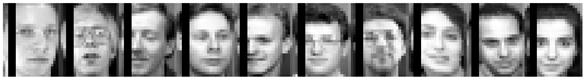Figure 2. Some images with SMV missing data.

Table 3. Experimental results with SMV missing data.

 5%data missing 10% data missing Time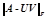IterationTimeIterationModel1 128.28 49.529 2661 0.2292 122.52 48.306 2488 0.2218 Model2 105.61 69.085 2156 0.2261 85.72 93.072 1774 0.2166 Model3 153.11 49.528 2664 0.2289 142.24 48.306 2488 0.2208
 25% data missing 40% data missing TimeIterationTimeIterationModel1 137.46 49.729 2785 0.1674 81.90 37.979 1795 0.3731 Model2 125.62 68.982 2599 0.1665 37.75 199.394 796 0.3646 Model3 158.85 49.729 2784 0.1670 100.97 37.979 1800 0.3620
 70% data missing TimeIterationModel 1 92.56 22.8694 1961 0.6225 Model 2 25.22 242.5778 520 0.5703 Model 3 103.07 22.8855 1850 0.5639

Table 4. Sparseness with SMV missing data.

 0% 5% 10% 25% 40% 70% Model 1 0.4936 0.4163 0.4248 0.4190 0.5798 0.7409 Model 2 — 0.4148 0.4235 0.4186 0.5726 0.7215 Model 3 — 0.4153 0.4228 0.4180 0.5658 0.7102

4.2.2. Analysis of Experiment Result

As illustrated in Table 3 and Table 4, the local feature approximate error between original data and data with missing data becomes more with the increase of the missing ratio, as well as the iteration  times, which means the restore ability becomes weaker. An interesting phenomenon for SMV type data missing is that the iterate times and the results of Model 1 and Model 3 is the same, this is because the position of the missing data is the same. For Model 2, the values at these position don’t attend the operation, which is the same as that for Model 1, so the M-step does not work in Model 3. For this type of missing data, Model 1 fits well. The sparseness of the local feature becomes more with the increase of the missing data.

4.3. Experiment 3

4.3.1. Experimental Results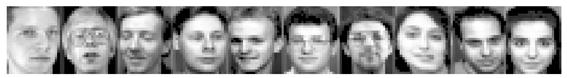Figure 3. Some images with SMS missing data.

Table 5. Experimental results with SMS missing data.

 5% data missing（value<21） 10% data missing（value<36） TimeIterationTimeIterationModel 1 134.18 51.0781 3127 0.0012 99.75 55.5599 2239 0.1204 Model 2 152.93 52.6064 3567 0.2175 172.21 55.4450 3650 0.2223 Model 3 154.36 45.9197 2711 0.2226 139.96 48.7178 2455 0.2168
 20% data missing（value<60） 40% data missing（value<107） TimeIterationTimeIterationModel 1 102.51 63.9386 2361 0.1923 179.77 86.8741 2826 0.2217 Model 2 116.42 66.1317 2736 0.2142 96.71 104.9639 2721 0.2357 Model 3 152.75 45.9197 2711 0.2226 152.89 27.6253 2726 0.2327
 70% data missing（value<166） TimeIterationModel 1 57.83 103.0335 1185 0.3832 Model 2 93.27 168.9273 2634 0.2567 Model 3 252.44 11.8293 4544 0.2424

Table 6. Sparseness with SMS missing data.

 0% 5% 10% 20% 40% 70% Model 1 0.4936 0.4082 0.4224 0.4321 0.5028 0.6002 Model 2 — 0.3799 0.3742 0.3505 0.2780 0.2373 Model 3 — 0.3802 0.3743 0.3743 0.2680 0.2205

4.3.2. Analysis of Experiment Results

As illustrated in Table 5 and Table 6, Model 2 costs less iterate time than that of Model 1 and Model 3, the iterate time for Model 1 and Model 3 is almost the same. As for the approximate error, Model 1 and Model 3 is similar. They are better than Model 2. The sparseness for Model 1 becomes bigger with the increase of missing data, which means that the local feature becomes less. The sparseness of Model 2 and Model 3 becomes bigger with the increase of the missing data, which means that the restore ability becomes weaker.

4.4. Experiment 4

4.4.1. Experimental Result

Table 7. Recognition rate for different models when 20% data missing.

 Original data Model 1 Model 2 Model 3 Not missing 87% —— —— —— RMV —— 64% 70% 71% SMV —— 70% 72% 72% SMS —— 62% 63% 66%

4.4.2. Analysis of Experiment Results

As illustrated in Table 7, the recognition rate of data without missing is more than that of data with missing data. Under the same ratio of the missing data, the restore ability of Model 3 is better than Model 2, and Model 2 is better than Model 1. The type of the missing data affects the recognition rate, the recognition rate for SMV data missing is better because the image with SMV missing data lost less local features.

5. Conclusions

This paper studied three local features extraction models in case of the training set has missing data basing on nonnegative matrix factorization algorithm: interpolation NMF model, weighted NMF model, and expectation-maximization NMF model. Three models are derived based on non-negative matrix factorization iterative formula. This paper compares the computational cost of three models. The second contribution of the paper is the classification of the missing data for image pixel values. We compared the local feature ex-traction ability of three modes by virtue of numerical experiments. Further research problem includes fast convergent algorithms, initial point scheme and different objective function will be considered in the future.

Acknowledgement

This work is supported by National Natural Science Foundation of China (No.11241005) and Yuncheng University Youth Foundation granted YQ-2012020.

References

1. Hongli Yang. Nonnegative matrix and tensor factorization and their applications. Ph.D thesis,2011.
2. Y.D.Kim and S.Choi. Weighted nonnegative matrix factorization. ICASSP 2009:1541-1544.
3. H.Lee,J.Yoo and S.Choi. Semi-supervised nonnegative matrix factorization IEEE Signal Processing Letters,2010,Vol(17)(1):4-7.
4. S.Zhang W.H.Wang,J.Ford and F.Makedon. Learning from incomplete ratings using nonnegative matrix factorization. SIGCOMM 2006:267-278.
5. E.Acar,D.M.Dunlavy,T.G.Kolda,and M.Morup. Scalable tensor factorization with missing data. Proceedings of the 2010 SIAM International Conference on Data Mining,2010.
6. N.Srebro,T.Jaakkola. Weighted low rank approximation.IMCL2003:720-727.
7. P.Paatero. Least squares formulation of robust, nonnegative factor analysis. Chemometrics and Intelligent Laboratory Systems,1997,Vol(37)(1):23-35.
8. A.M.Buchanan and A.W.Fitzgibbon. Damped Newton algorithms for matrix factorization with missing data CVPR2005,Vol(2):316-322
9. V.D.Blondel,N.D.Ho,and P.V.Dooren. Weighted nonnegative matrix factorization and face feature extraction. Image and Vision Comput-ing,2008.
10. G.Tomasi and R.Bro.Parafac and missing values. Chemometrics and intelligent laboratory systems.2005,Vol(75)(2):163-180.
11. A.P.Dempster,N.M.Laird and D.B.Rubin. Maximum likelihood from incomplete data via the EM algorithm. Journal of Royal Statistical Society,1977,Vol(39)(1):1-38.
12. E.J.Candes and Y.Plan. Matrix completion with noise.arXiv:0903.3131v1vl,2009.
13. E.J.Candes and T.Tao.The power of convex relaxation: near-optimal matrix completion.arXiv:0903.1476vl,2009.
14. K.R.Gabriel and S.Zamir. Lower rank approximation of matrices by least squares approximation with any choice of weights.Technimetrics,1979,Vol(21)(4):489-498.
15. A.Ruhe. Numerical computation of principal components when several observations are missing. Technical report, University of Umea,Sweden,1974.
16. R.J.A.Little and D.B.Rubin. The analysis of social science data with missing values. Sociological Methods and Research,1989,Vol(18)(2-3):292-326.
17. R.L.Carter. Solutions for missing data in structural equation modeling. Research and Practice in assessment,2006,Vol(1)(1):1-6.
18. D.Guillamet,J.Vitria and B.Schiele. Introducing a weighted nonnegative matrix factorization for image classification. Pattern Recognition Letters,2003,Vol(24)(14):2447-2454.
19. X.Li and K.Fukui. Fisher nonnegative factorization with pair wise weighting.MVA 2007,IAPR:380-383.
20. P.J.B.Koeck. Missing data in image and signal processing: the case of binary objects. International journal for light and electron optics,2004,Vol(115)(10):459-472.
21. R.B.Kline. Principles and practices of structural equation modeling. Third edition, John Wiley &Sons, Inc., New York,1988.
22. D.D.Lee and H.S.Seung. Learning the parts of objects by nonnegative matrix factorization. Nature,1999,Vol(401)(6755):788-794.

 Contents 1. 2. 3. 3.1. 3.2. 3.3. 3.4. 4. 4.1. 4.2. 4.3. 4.4. 5.
Article ToolsAbstractPDF(816K)